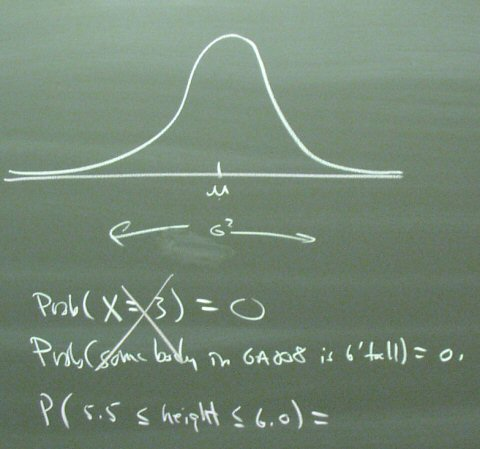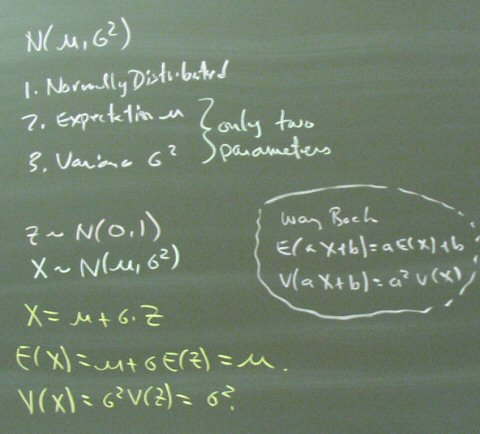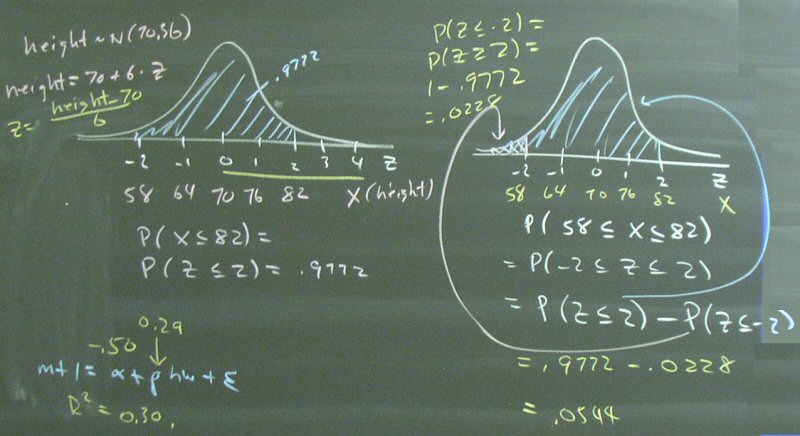## October 28, 2003

### Homework

Ch 6, #9,10,11,12,14,16,20. Due 10/30.
Ch 6, #28,30. Due 11/4.
Ch 6, #39,40,42,43,44,55. Due 11/11.

We did not get to the lecture material on 11/4 so the due date for the associated problems is now 11/11.

Ch. 7, 4,6,8,10,14,22,24,26,28,30. Due 11/13.
Ch. 8, 22,23,24,26,28. Ch 9, 5,6,7,20,22,24,26. Due 11/18. No PHStat, please.

Posted by bparke at 11:39 AM

### The Normal Distribution

The normal distribution is important primarily because the Central Limit Theorem says that averages tend to be normally distributed. We will get back to that point, but for now we are concentrating on learning how to calculate probabilities for normal distributions.Continuous-valued random variables have a probability of zero of taking on any particular value. That is, nobody in class is precisely six feet tall. The relevant concept is the probability that a continous-valued random variable is within an interval.The basic mechanism will be to work with a standard normal variable that has probabilities in the table in the back of the book. We will get very good at changing scales.Our first examples set the tone for the homework. Take a look at the homework problems and see if these diagrams help. We will certainly draw more diagrams.Posted by bparke at 11:22 AM

### Midterm 1

MT 1 came back today. An answer sheet is available. If you have a grading problem, I take written appeals only. The exam and homework scores are here.

Posted by bparke at 11:11 AM